Function Repository Resource:

# CombinatorFixedPoint

Evolve a combinator expression to its fixed point based on defined rules and evaluation scheme

Contributed by: Wolfram Research
 ResourceFunction["CombinatorFixedPoint"][cmb] evolves combinator expression cmb to its fixed point using S and K combinator transformation rules and single leftmost outermost updating order. ResourceFunction["CombinatorFixedPoint"][cmb,scheme] evolves cmb to its fixed point using S and K combinator transformation rules in matches found by scheme at each step. ResourceFunction["CombinatorFixedPoint"][rules, cmb,scheme] evolves cmb to its fixed point using rules in matches found by scheme at each step.

## Details and Options

The input cmb must be given as a combinator expression in nested bracket form.
If scheme is specified, direction ("Leftmost", "Rightmost"), depth order ("Outermost", "Innermost") and number of matches (integer number or Infinity) must be specified, in order of their priority as sorting criteria.
The combinator transformations of interest should be given as a list of Rule or RuleDelayed elements in rules.
ResourceFunction["CombinatorFixedPoint"] takes the following options:
 MaxSteps Infinity maximum steps to take in evolving cmb "MaxSize" Infinity maximum leaf count allowed for evolution of cmb "SKGlyphs" symbols used to specify default transformation rules

## Examples

### Basic Examples (2)

Calculate the fixed point of a combinator expression using the default single leftmost outermost update with S and K combinator transformation rules:

 In:=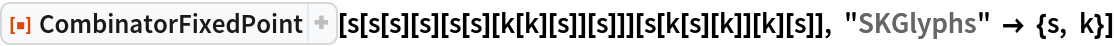Out=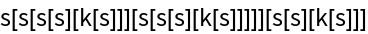Specify the update scheme and only use the K combinator transformation rule to evolve the combinator expression to its fixed point:

 In:=Out=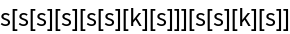### Properties and Relations (2)

Calculate the fixed point of a combinator expression using the default single leftmost outermost update with S and K combinator transformation rules:

 In:=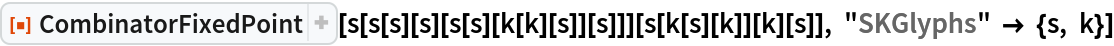Out=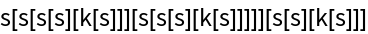One can replicate this result with the use of ReplaceAll in FixedPoint:

 In:=Out=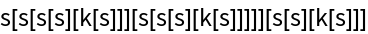### Possible Issues (1)

The "MaxSize" and "MaxSteps" options can be useful for avoiding excessively long combinator evolutions, but will throw failures if their values are surpassed:

 In:=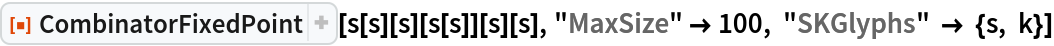Out=In:=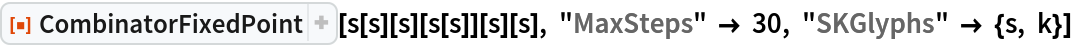Out=## Version History

• 1.0.1 – 05 December 2020
• 1.0.0 – 04 December 2020